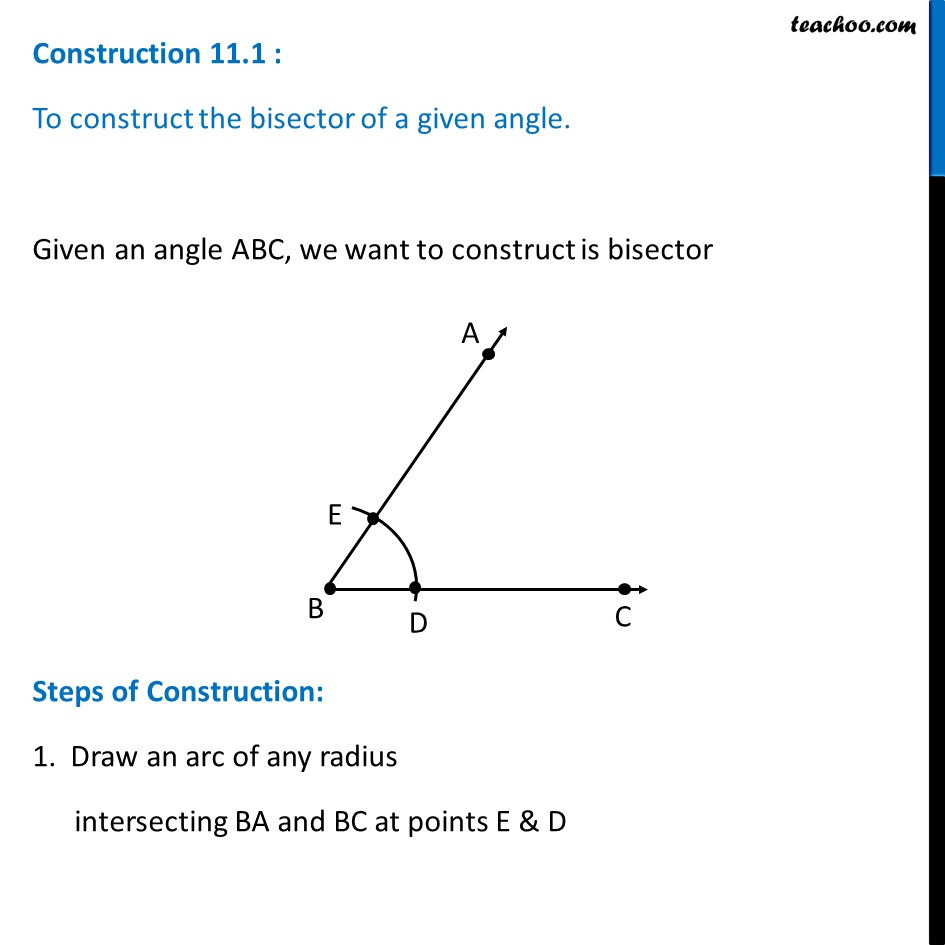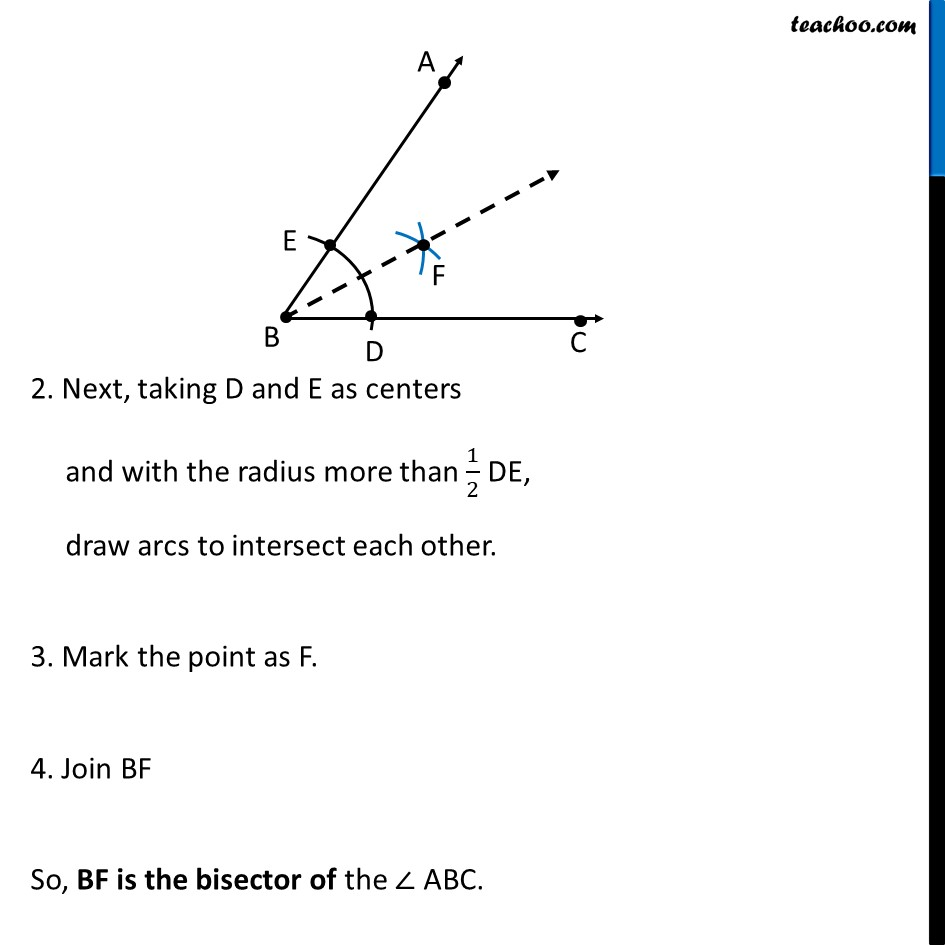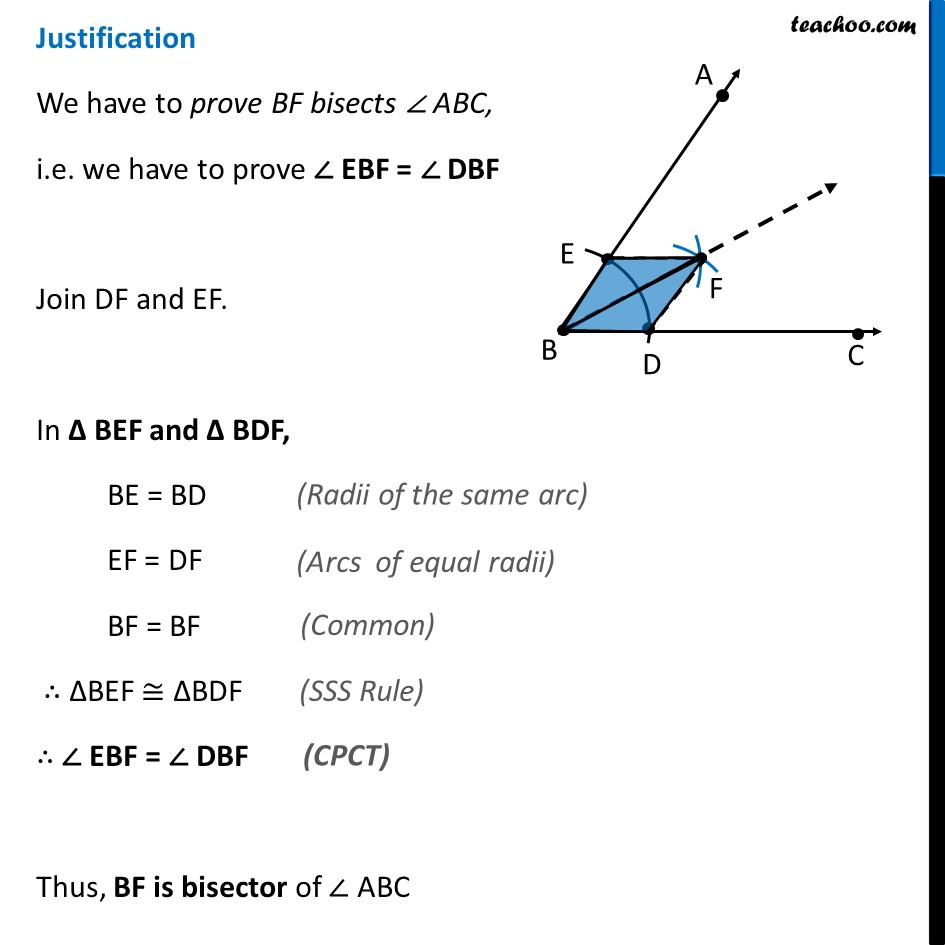1. Chapter 11 Class 9 Constructions
2. Concept wise
3. Constructing bisector of angle and perpendicular bisector

Transcript

Construction 11.1 : To construct the bisector of a given angle. Given an angle ABC, we want to construct is bisector Steps of Construction: 1. Draw an arc of any radius intersecting BA and BC at points E & D 2. Next, taking D and E as centers and with the radius more than 1/2 DE, draw arcs to intersect each other. 3. Mark the point as F. 4. Join BF So, BF is the bisector of the ∠ ABC. Justification We have to prove BF bisects ∠ ABC, i.e. we have to prove ∠ EBF = ∠ DBF Join DF and EF. In Δ BEF and Δ BDF, BE = BD EF = DF BF = BF ∴ ∆BEF ≅ ∆BDF ∴ ∠ EBF = ∠ DBF Thus, BF is bisector of ∠ ABC (Radii of the same arc) (Arcs of equal radii) (Common) (SSS Rule) (CPCT) (CPCT)

Constructing bisector of angle and perpendicular bisector